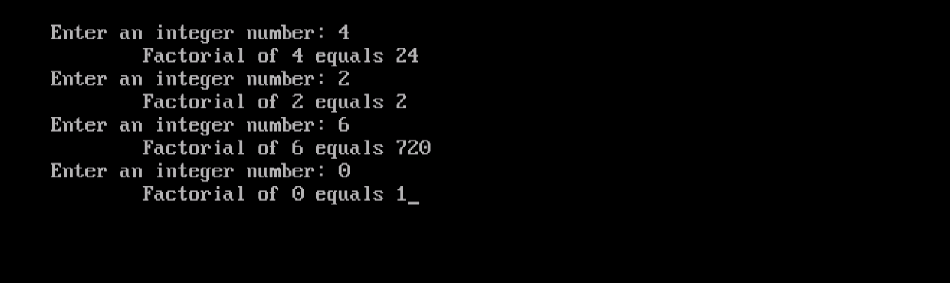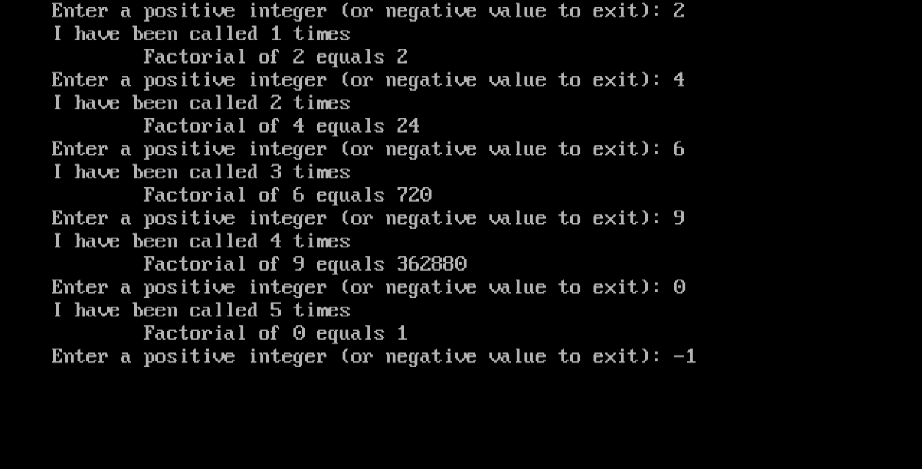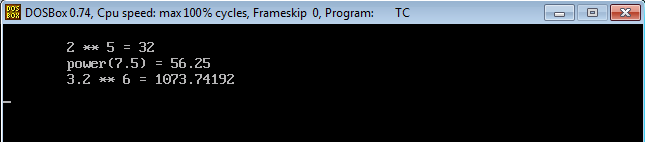Continuing with Functions from Part one, here is Part two.

Recursion
A user-defined function can call itself. Yes, you have read it correctly, and there are no typos. Within the function body, a call to the function can be done. Such recursive calls are usually controlled by a condition. For a recursive function to work correctly, the function must converge at some point, in a way that makes the condition evaluate to false, hence ending the recursive calls, and the function.

Example
Let’s re-write the factorial program using the recursive function method.
Consider the following code:

```#include<iostream.h>
#include<conio.h>
long factorial(int x)
{
if(x==1 || x==0)
return 1;
else
return x * factorial(x-1);
}
void main()
{
int num;
cout << "\nEnter an integer number: ";
cin >> num;
if (num < 0)
{
cout<<"**** WRONG INPUT ****\n";
getch();
}
else
{ cout << "\tFactorial of " << num << " equals " << factorial(num);
getch();
}
}
```

Here’s the output after executing the program four times.Overloading means having two or more functions with the same name. Assigning the same name to several functions is not because we have a shortage in names, but because they all do the same job, and it would be easier to give them all the same name.

The question now arises: when an overloaded function is called within a program, how will the compiler insert the code of the right (intended) function? How could the compiler distinguish between the functions?!

The compiler uses something called the function signature to distinguish between functions defined with the same name. The signature of a function consists of the number of arguments it expects, and their data types.

To understand the idea, consider we have a function named func_new(). The following overloaded function definitions are legal in C++:

```func_new()
{
Function Body
}

func_new(int x)
{
Function Body
}

func_new(float y)
{
Function Body
}

func_new(int z, char ch)
{
Function Body
}
```

Variable Scopes
The scope of a variable defines its range of visibility. In terms of scope, variables can be divided into two main types:

• Local variables.
• Global Variables.

Local variables, in turn, are divided into two main sub-types: local, and static local. In this section, we are going to investigate those types.

Local Variables
Inside a function, any function, either the main(), or any other user-defined function, when a variable is defined like this:

```int num;
float x;
long result;
```

That variable is said to be local. Being local means the variable is only visible inside the code block in which it was declared. Its life is limited to the lifetime of a function call. Each time a function is called, the variable is declared. When the function returns, the variable is destroyed.

All variables we used in the programs, written so far in this series are examples for local variables.
If not explicitly initialized by an assignment statement, the C++ compiler will not initialize a local variable. So, an un-initialized local variable could have any initial garbage value.

Static Local Variables
A static variable is a special case of local variables. A static variable is still seen only inside the function in which it was defined, but it will live from the moment its function is first called until the program exits.

To mark a variable as static, all we need to do is to add the word static before its declaration.

```static int counter;
```

When a static variable is initialized, the initialization will occur only once. In subsequent function calls, the variable will not be re-initialized.

When the function returns, static variables will not be destroyed (as in ordinary local variables). They retain their values, so if the function gets called again, the static variable are available with their values. Static variables stay alive until the program exits.Global Variables
Global variables are defined outside the program functions, so, they are not bound or limited to a specific function. Consequently, a global variable is seen to all functions of the program, and its lifetime is the lifetime of a program. Global variables are usually declared just after the #include statements at the beginning of the program. If a global variable is not explicitly initialized, it will be set to 0.

Example
In this example, we will modify the Factorial program, so that every time the factorial function is called a message is displayed to the user: “I have been called n times”.

Consider the following code:

```#include<iostream.h>
#include<conio.h>
long factorial(int x)
{
static int count=0;
long result=1;
for(int i=x;i>1;i--)
result*=i;
cout << "I have been called "<< ++count << " times\n";
return result;
}
void main()
{
clrscr();
do
{
int x;
cout << "Enter a positive integer (or negative value to exit): ";
cin >> x;
if (x<0) break;
cout << "\tFactorial of " << x << " equals " << factorial(x) << endl;
}
while (1);
}
```

When executed, it should print the required message each time the factorial function is called.Optional (Default) Arguments
C++ gives us the capability to provide functions with default values for their arguments. Consider a function that accepts three arguments: one, two, or the three arguments could have default values. When the function is called, if an argument with default value is missing, the default value is used.

Note
The optional arguments must come after all the non-optional (mandatory) arguments in the argument list of a function.

Example
In this example, we are going to write a function that calculates the result of raising a float number to the power of an integer number. The function accepts two arguments the base (float), and the exponent (integer). If the exponent wasn’t provided, the function should consider a default value of 2.

Consider the following program:

```#include<iostream.h>
#include<conio.h>
double power (float base, int exp=2)
{
double result = 1;
for (int i=1; i<=exp;i++)
result *= base;
return result;
}
void main()
{
clrscr();
cout << endl;
cout << "\t2 ** 5 = " << power (2,5) << endl;
cout << "\tpower(7.5) = " << power (7.5) << endl;
cout << "\t3.2 ** 6 = " << power (3.2, 6) << endl;
getch();
}
```

Let’s see how this will execute:Great!!

Summary
That was part two in the Functions topic.

• A function can call itself. This should be well-controlled, otherwise, the function won’t converge, and the program will crash.
• Function Overloading means more than a function could all have the same name, given that the number of arguments and / or the arguments data type is different.
• In terms of scope, variables can be classified as local and global. Local variables include a special type called static variables.
• Arguments could have default values. An argument with default values is said to be an optional argument.

The next article will talk about Structure and Enums; A topic that you shouldn’t miss. So, see you there.

### Top 10 cyber security threats that arose last year

21,501Fans
4,218Followers
103,000Subscribers

### Top 10 cyber security threats that arose last year

- Advertisement -